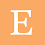Macro Economics Theory Mcqs - Study For Buddies

## Sunday, April 17, 2022

S.Y B.COM
SEMESTER - 3

MACRO ECONOMICS THEORY
(MET)
MCQS: 19 JANUARY 2022

1. While we calculate National Income through Value Added method, we do not include one of the following components:

A. Purchase of second hand goods
B. Imputed rent values of self occupied houses
C. Value of Production for self
consumption
D. Net Factor Income from Abroad

2. In consumption line C=a+ b.Y, what does 'b' denote?

A. Slope of consumption curve
B. Multiplier of Income
C. Marginal Propensity to Save
D. Average Propensity to Consume

3. Which of the following do economists view as investment spending?

A. Spending on physical capital
B. Stocks
C. Bonds
D. Mutual fund investing

4. National Income at Factor Cost can be defined as:

A. Net National Product - Net Indirect
Taxes
B. Gross National Product - Net
Indirect Taxes
C. Net National Product - Total
Indirect Taxes
D. Net National Product - Subsidies.

5. In classical economics sense Y=C+I can be re written as:

A. Y=C+S
B. Y=S+I
C. Y+C =S
D. Y+I=S+I

6. The marginal propensity to consume is defined as ____________.

A. The change in consumer spending
divided by the change in aggregate
disposable income.
B. The change in saving minus change
in investment.
C. The proportion of total disposable
income that the average family
consumes.
D. The change in consumer spending
minus the change in aggregate
disposable income.

7. Suppose that the aggregate consumption function is given by the equation: C = 200+0.8YD, where C represents consumption and YD represents disposable income. If disposable income is Rs. 500, aggregate consumption is:

A. Rs. 600
B. Rs. 200
C. Rs. 500
D. Rs. 400

8. While we calculate National Income through Expenditure method, we should include one of the following components:

A. Private expenditure on goods and
services
B. Purchase of Shares and Bonds
C. Transfer Payments
D. Expenditure on Intermediate Goods

9. When a government adopts a balance budget policy it spends only as much as it collects through

A. taxation
B. exports
C. bonds
D. foreign direct investments

10. Classical economists treated money as _____________.

A. Medium of Exchange
B. Store of value
C. Means of deferred payment
D. Measure of Value

11. If NDP at factor cost is Rs. 210 million and Indirect taxes, Rs. 51 million, GNP at Market prices is Rs. 610 million, the value of NDP at market prices will be Rs. __________ million.

A. Rs. 261 million
B. Rs. 820 million
C. Rs. 271 million
D. Rs. 539 million

12. If for an economy, GDP at market Prices is Rs. 3094 million, Net Indirect Taxes is Rs. 564 million and Capital consumption allowance is Rs. 342 million, the value of GDP at factor cost would be Rs. _________ million

A. Rs. 2530 million
B. Rs. 4000 million
C. Rs. 3436 million
D. Rs. 3658 million

13. 'An individual will usually see a 10% cut to nominal wages as unfair, but the same proportion of price rise would not be fiercely contested'. The statement is best reflected in the following terms:

A. Money Illusion
B. Price Illusion
C. Output Illusion
D. Income Illusion

14. In a simple model of Keynes income determination, import is assumed to be a function of the following (identify correct ones)
I. Autonomous Import
II. National Income
III. Investment
IV. Government Expenditure

A. I & II
B. I, II & III
C. II, III & IV
D. II & III

15. 'Saving is good for individuals but not so good for the society as a whole as it ends up in lowering standard of living, income, and output'. This phenomena is called ____________.

B. Paradox of Wage and employment
C. Paradox of Income and Saving

16. In the context of classical macro economics, the demand and supply of labour together can determine the following:

A. Real Wages, Employment and Real Output
B. Real Wages and Real Unemployment
C. Nominal Wages, Nominal Employment and Nominal Output
D. Production function, Income and Output

17. The rational expectation theory is different from Keynesian Theory in the sense that ____________.

A. Whatever happens in the economy cannot be correctly predicted by the people
B. Involuntary unemployment cannot prevail
C. Producers and consumers adjust their behaviour rapidly.
D. Producers and consumers are able to collect necessary information

18. Keynesian explanation of a deficiency in aggregate demand originated from __________.

A. Real time experience of Great Depression
B. Severe inflation in the post war situation
C. Say's Law of Market
D. Pigou's explanation of wage flexibility.

19. If we apply Say's Law of Market in a monetized economy we would be closer to explain ____________.

A. Two Sector Circular Flow Model
B. Marginal Productivity Theory of Wages
C. Principle of Effective Demand
D. Wage Price rigidity.

20. The national income under four sector model is less than that of under three sector model because ____________.

A. Import is also function of national income
B. Import is autonomous
C. Export is autonomous
D. Export is also function of national income

22. One of the following is not a key feature of Keynesian Theory

A. Rate of Interest is a reward for saving
B. Under employment is a normal situation in the economy
C. Wages are rigid downwards and flexible upwards
D. There is only indirect relationship between money supply and price level.

1.